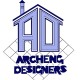# Design of Singly reinforced sections | Design Method 2

#### Guide to design of Singly reinforced Sections

For “Singly reinforced sections” article series, we have covered the following:

Now we will move on with our discussion on “2nd Design method” for the design of Singly reinforced Sections.
We will follow a simple three-step procedure for the design of singly reinforced sections.

#### Step One:

Given that:

• Dimensions of section (b and d)
• Area of tensile steel (Ast)
• Modular ratio (m)

From the figure above, we can see that the neutral axis is situated at the centre of gravity of a given section. Therefore, the moments of area on either side are equal.

Therefore, moment of area on compression side = moment of area on tension side

Moment of area on compression side = Area of compression side x distance of c.g. of compression area from N.A.

= (bx).(x/2)

= bx.x/2

#### Step Two:

Moment of area on tension side = equivalent area of concrete x distance of c.g. of tensile steel from N.A.

= (m Ast) x (d-x)

= mAst(d-x)

#### Step three:

Where, mAst = equivalent area of concrete

Therefore, bx.x/2 = mAst (d – x)

### 4 thoughts on “Design of Singly reinforced sections | Design Method 2”

1.i need rcc structure steel dezine for 45 * 90feet hall. Including
Beam & Coloum dezine . For singel story hall.

•Dear Hemant,

We do offer paid structural design services. Please email benruk@gmail.com for further info.

2.hi,this is very helpful sight and I have cleared many douts.I want to know about the designing of the foundation and columns.like assume a area of land and then how to design a foundation and columns(how many column to be needed with dimension) and what type of foundation should be laid.please clear these questions

•